# RS Aggarwal Solutions for Class 10 Maths Chapter 1 Real Numbers Exercise 1A

RS Aggarwal Solutions for Class 10 Maths Chapter 1 Real Numbers, contains solutions for all Exercise 1A questions. RS Aggarwal Class 10 Real Numbers Exercise 1A questions and answers help students to excel their skills and score good marks. Revise Class 10 Maths study material on real numbers which are prepared by BYJU’S subject experts. Download the RS Aggarwal Solutions of Class 10 and practice offline.

## Download PDF of RS Aggarwal Solutions for Class 10 Maths Chapter 1 Real Numbers Exercise 1A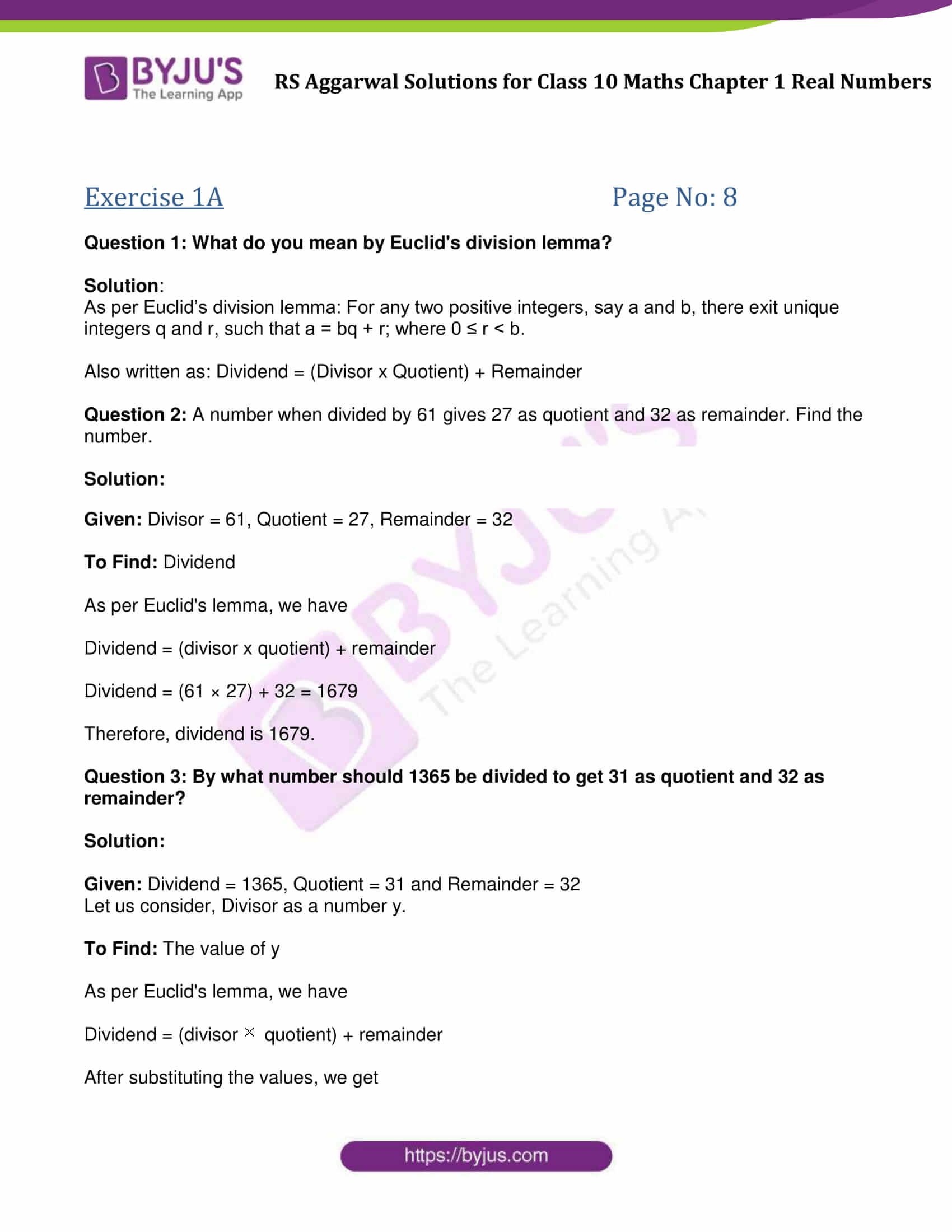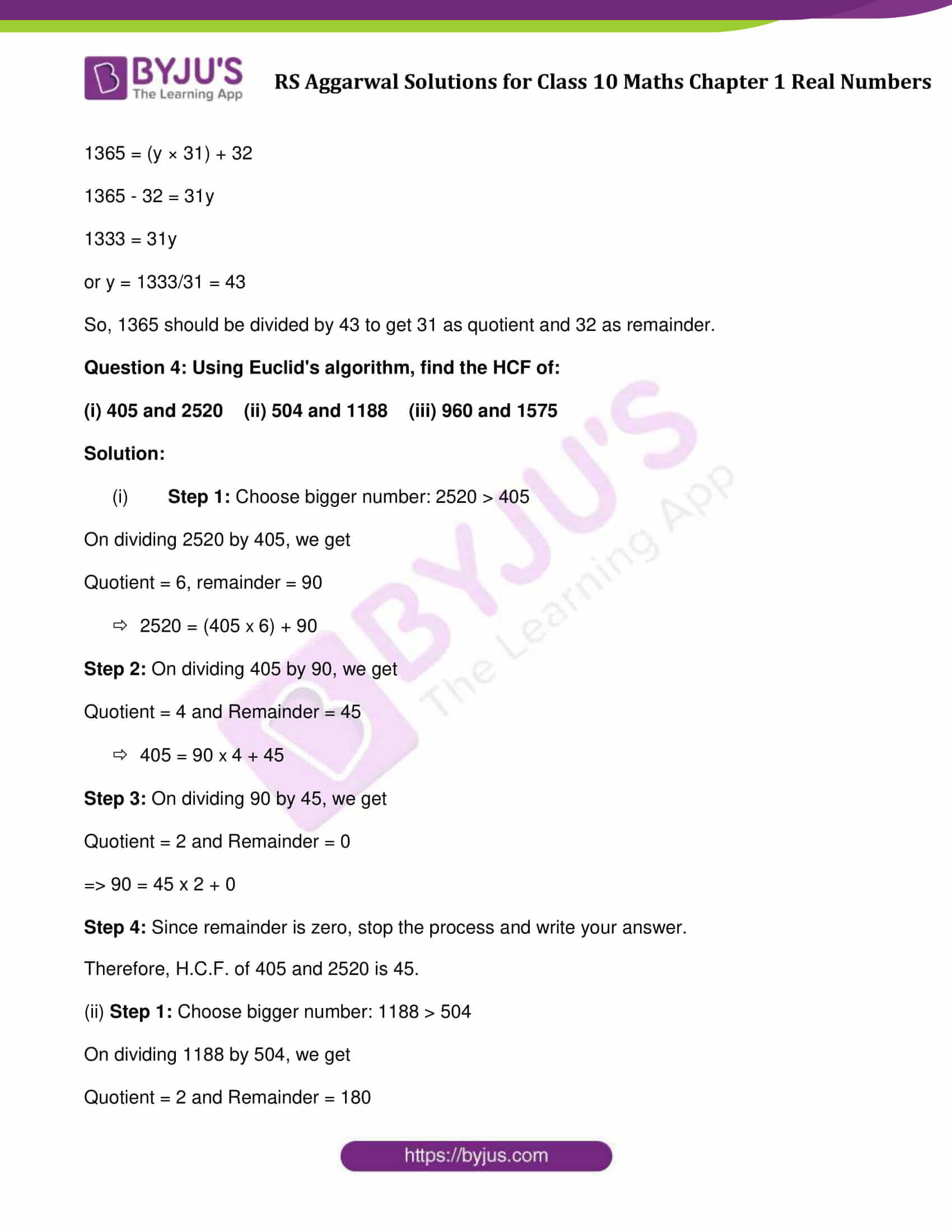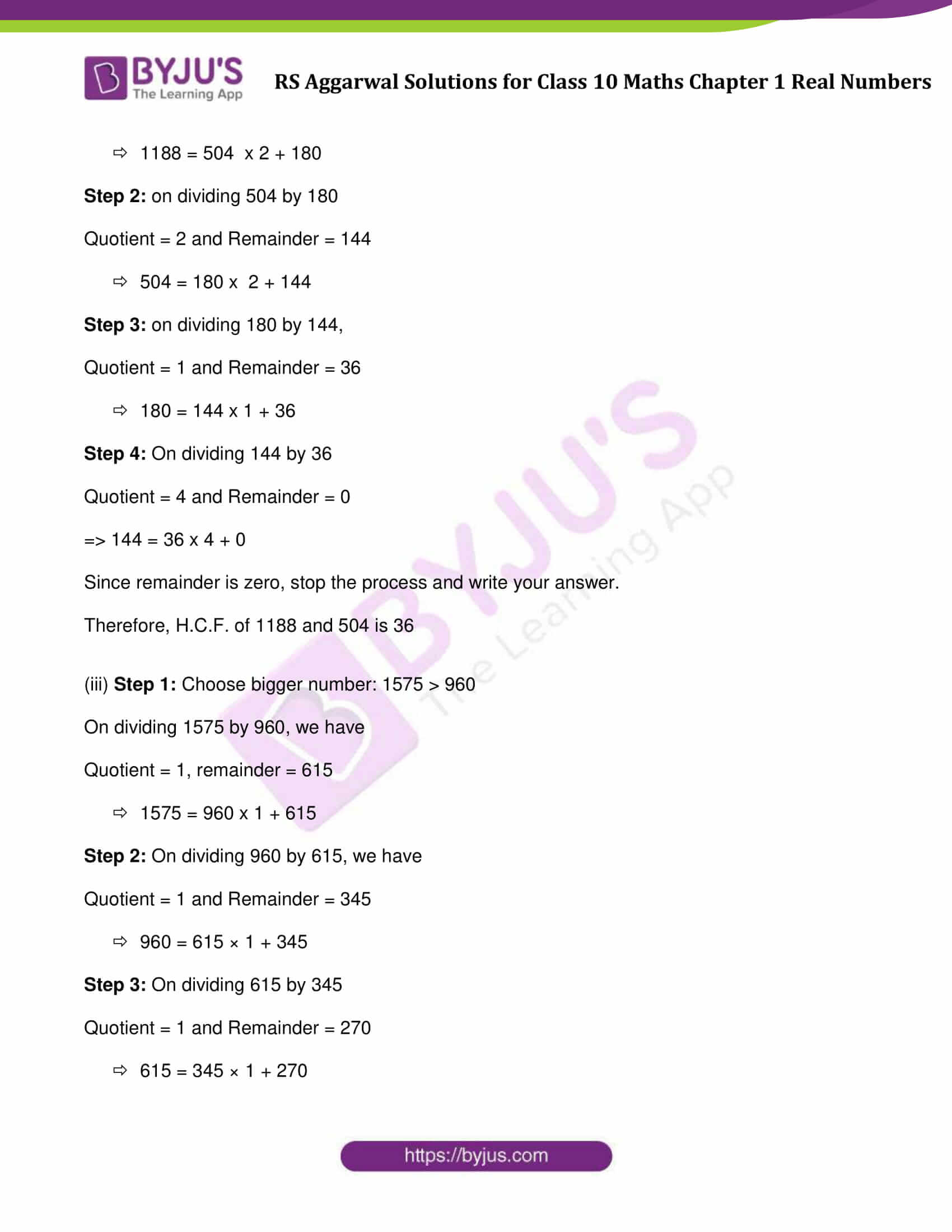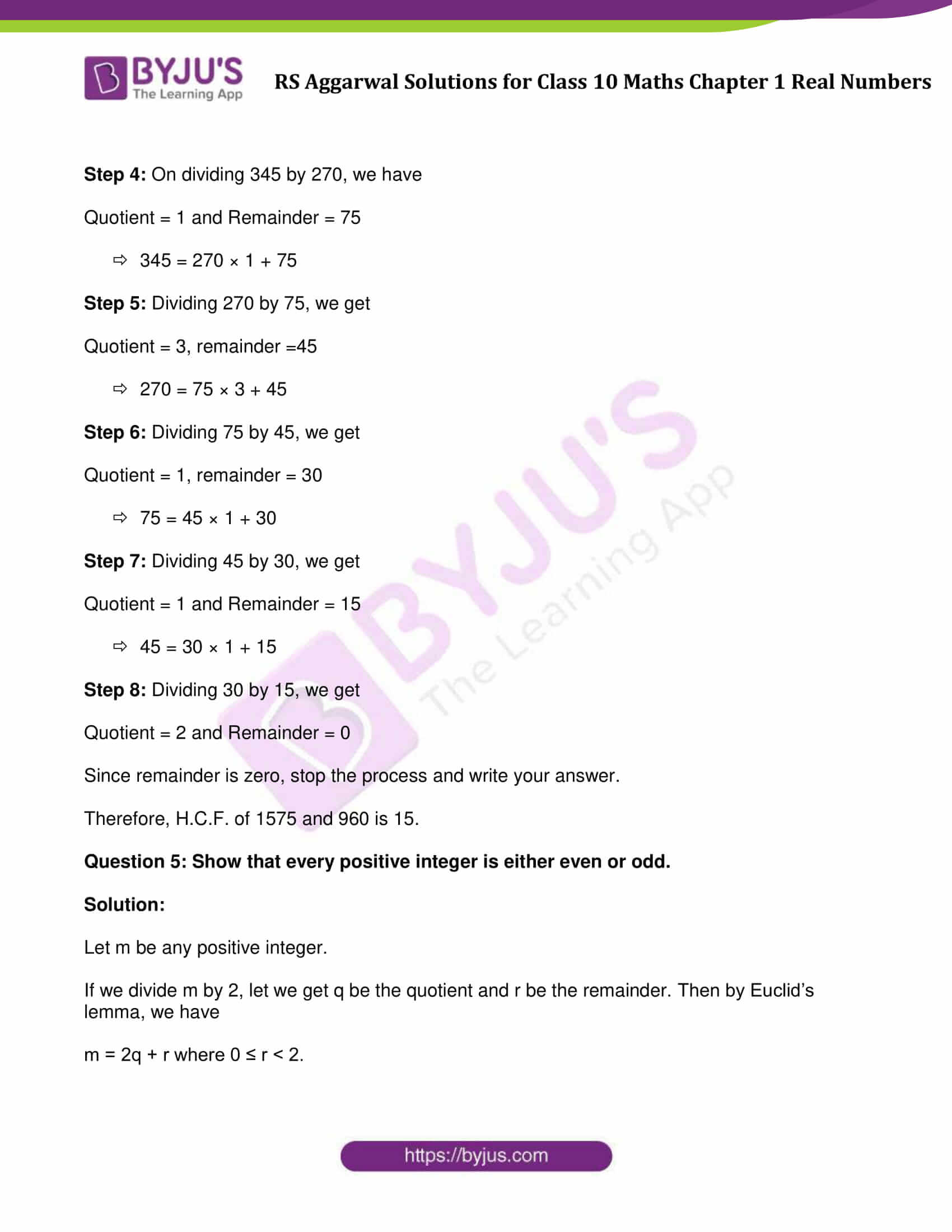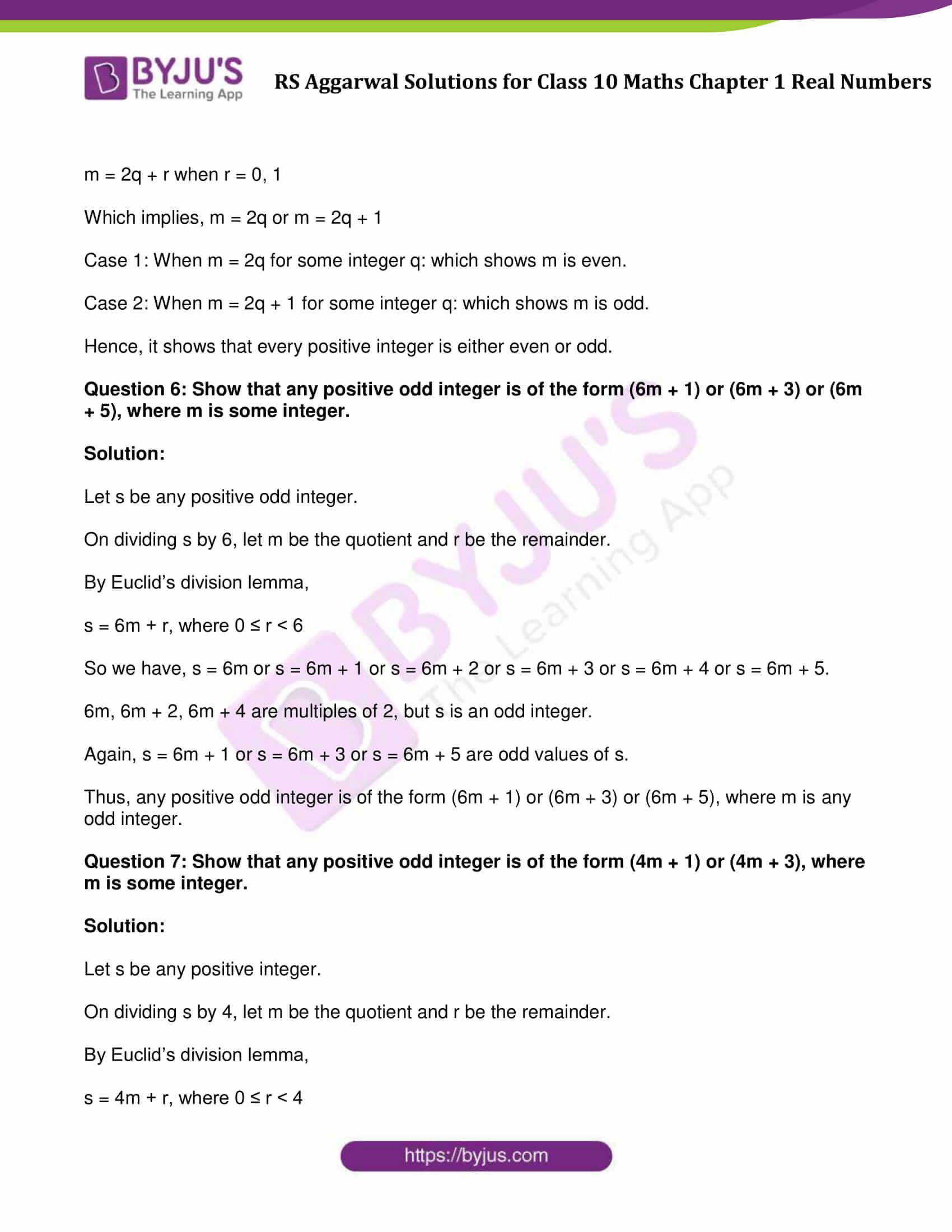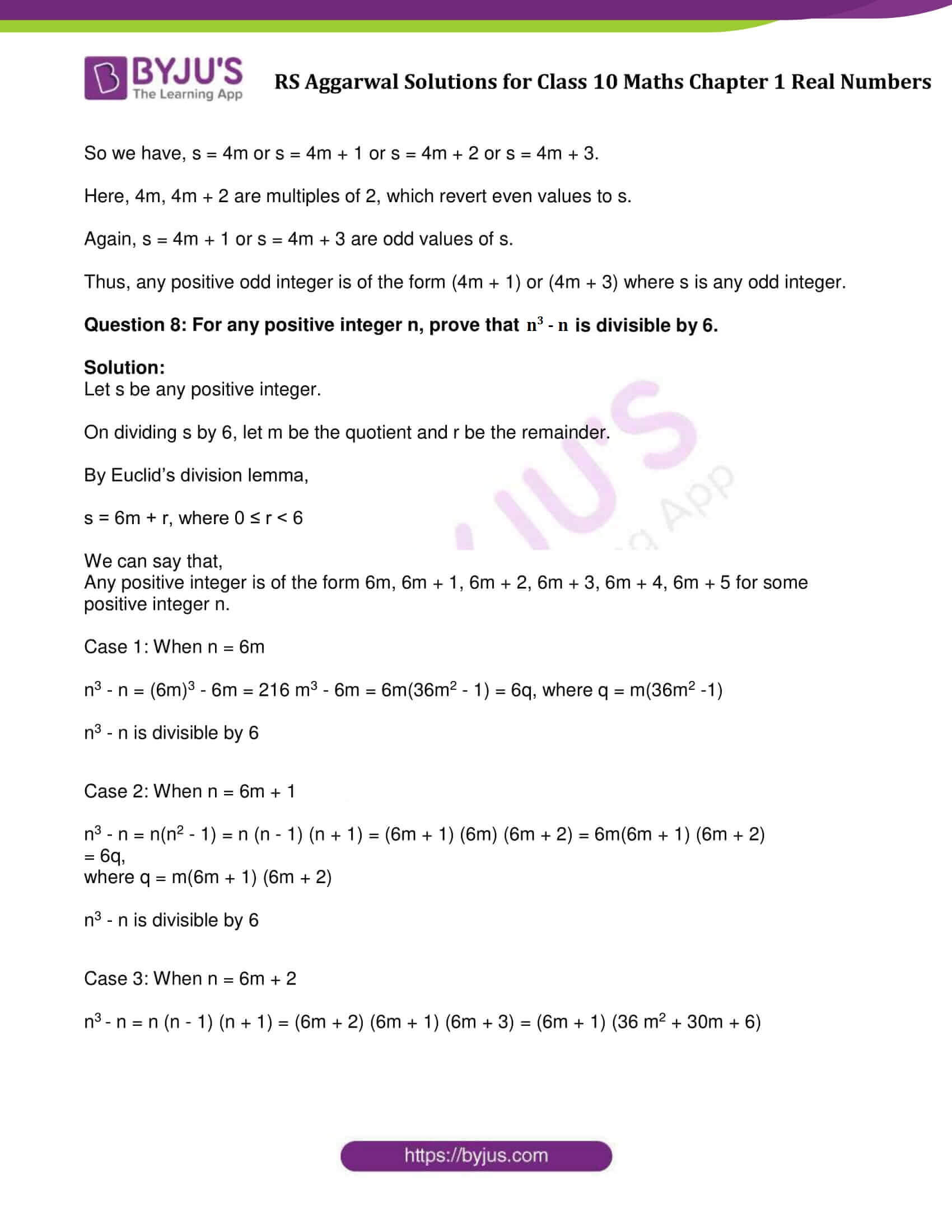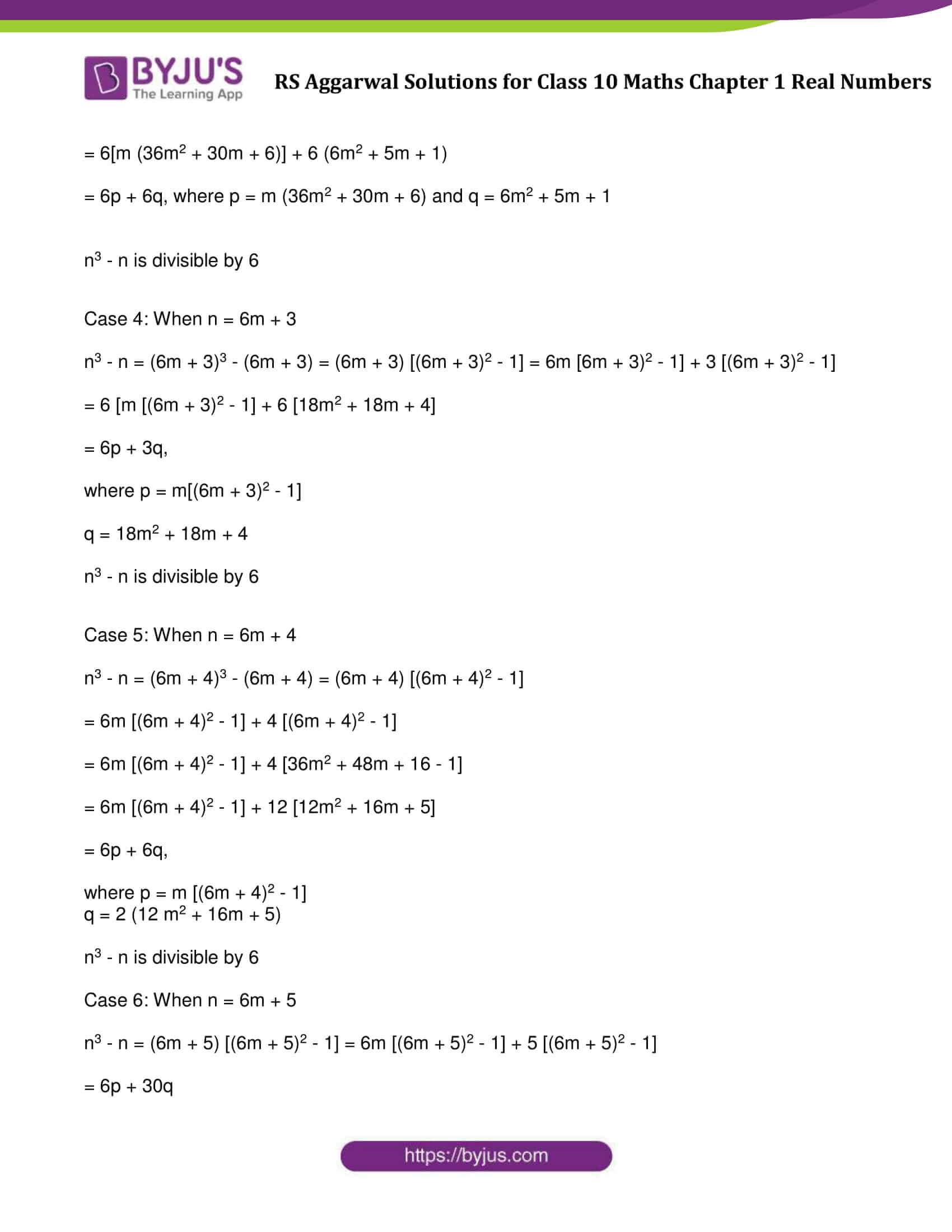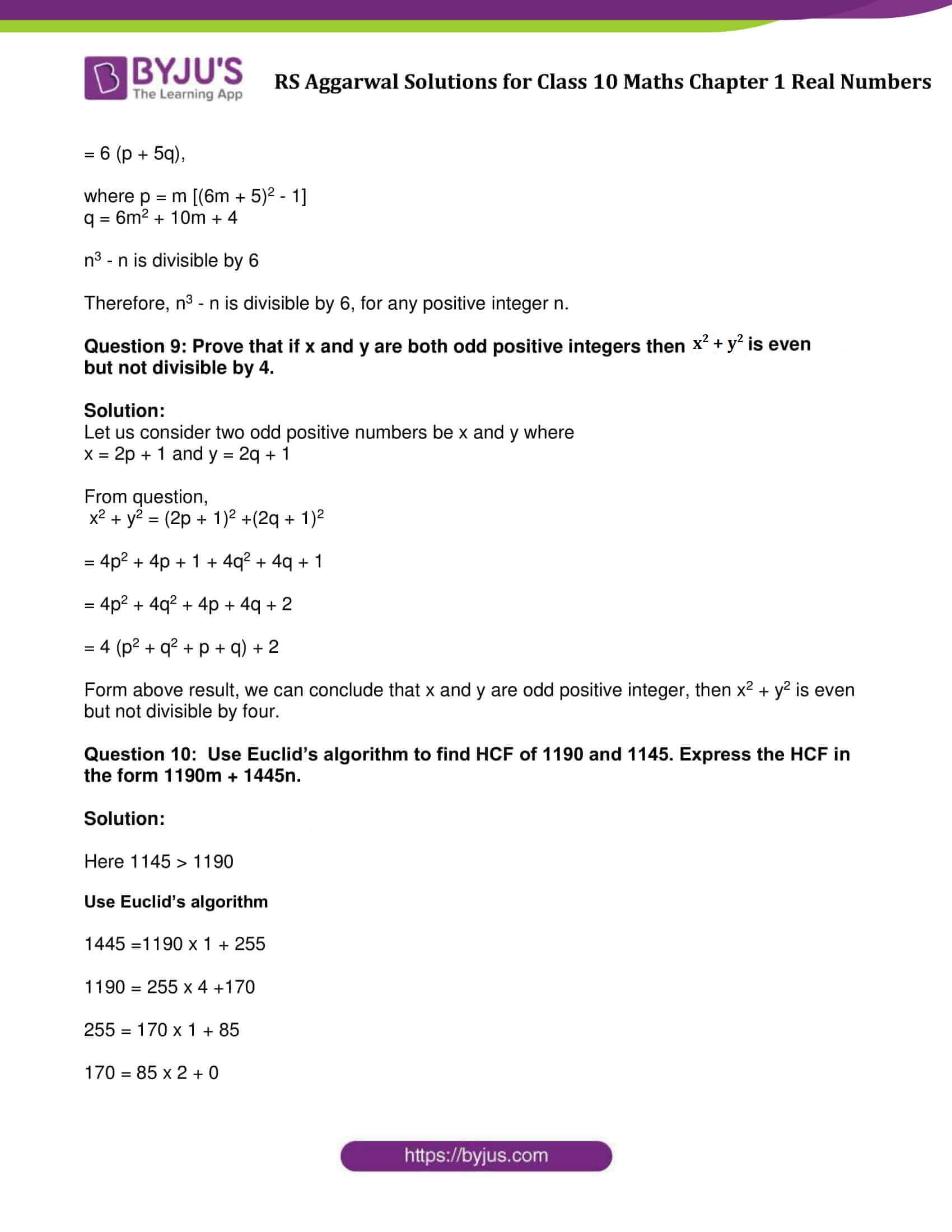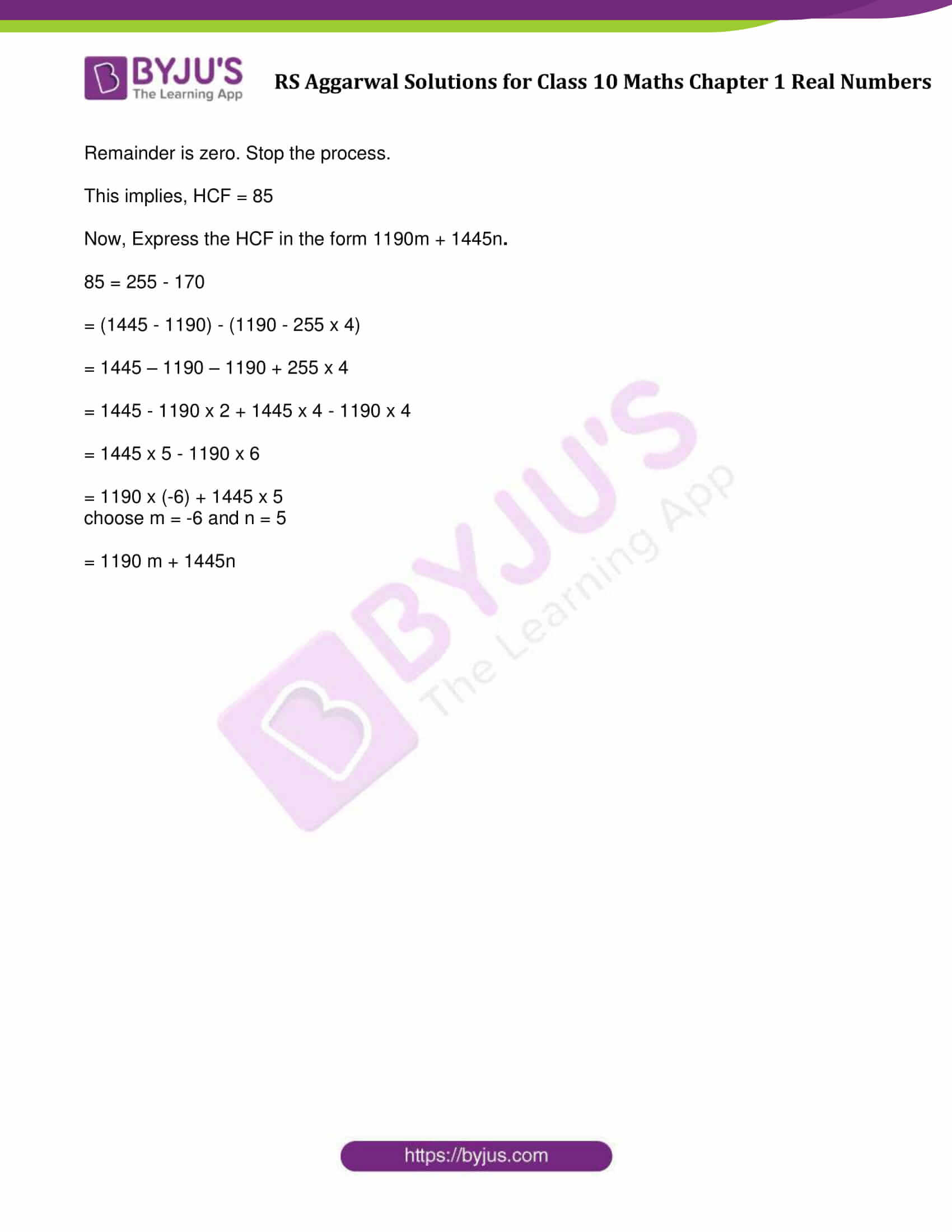### Access other exercise solutions of Class 10 Maths Chapter 1 Real Numbers

Exercise 1B Solutions : 27 Questions (Short Answers)

Exercise 1C Solutions : 3 Questions (Short Answers)

Exercise 1D Solutions : 11 Questions (Short Answers)

Exercise 1E Solutions : 23 Questions (Short Answers)

## Exercise 1A Page No: 8

Question 1: What do you mean by Euclid’s division lemma?

Solution:

As per Euclid’s division lemma: For any two positive integers, say a and b, there exit unique integers q and r, such that a = bq + r; where 0 ≤ r < b.

Also written as: Dividend = (Divisor x Quotient) + Remainder

Question 2: A number when divided by 61 gives 27 as quotient and 32 as remainder. Find the number.

Solution:

Given: Divisor = 61, Quotient = 27, Remainder = 32

To Find: Dividend

As per Euclid’s lemma, we have

Dividend = (divisor x quotient) + remainder

Dividend = (61 × 27) + 32 = 1679

Therefore, dividend is 1679.

Question 3: By what number should 1365 be divided to get 31 as quotient and 32 as remainder?

Solution:

Given: Dividend = 1365, Quotient = 31 and Remainder = 32

Let us consider, Divisor as a number y.

To Find: The value of y

As per Euclid’s lemma, we have

Dividend = (divisor  \times  quotient) + remainder

After substituting the values, we get

1365 = (y × 31) + 32

1365 – 32 = 31y

1333 = 31y

or y = 1333/31 = 43

So, 1365 should be divided by 43 to get 31 as quotient and 32 as remainder.

Question 4: Using Euclid’s algorithm, find the HCF of:

(i) 405 and 2520 (ii) 504 and 1188 (iii) 960 and 1575

Solution:

(i)

Step 1: Choose bigger number: 2520 > 405

On dividing 2520 by 405, we get

Quotient = 6, remainder = 90

⇨ 2520 = (405 x 6) + 90

Step 2: On dividing 405 by 90, we get

Quotient = 4 and Remainder = 45

⇨405 = 90 x 4 + 45

Step 3: On dividing 90 by 45, we get

Quotient = 2 and Remainder = 0

⇨ 90 = 45 x 2 + 0

Step 4: Since remainder is zero, stop the process and write your answer.

Therefore, H.C.F. of 405 and 2520 is 45.

(ii)
Step 1: Choose bigger number: 1188 > 504

On dividing 1188 by 504, we get

Quotient = 2 and Remainder = 180

⇨ 1188 = 504  x 2 + 180

Step 2: on dividing 504 by 180

Quotient = 2 and Remainder = 144

⇨ 504 = 180 x 2 + 144

Step 3: on dividing 180 by 144,

Quotient = 1 and Remainder = 36

⇨ 180 = 144 x 1 + 36

Step 4: On dividing 144 by 36

Quotient = 4 and Remainder = 0

⇨ 144 = 36 x 4 + 0

Therefore, H.C.F. of 1188 and 504 is 36

(iii)

Step 1: Choose bigger number: 1575 > 960

On dividing 1575 by 960, we have

Quotient = 1, remainder = 615

⇨ 1575 = 960 x 1 + 615

Step 2: On dividing 960 by 615, we have

Quotient = 1 and Remainder = 345

⇨ 960 = 615 × 1 + 345

Step 3: On dividing 615 by 345

Quotient = 1 and Remainder = 270

⇨ 615 = 345 × 1 + 270

Step 4: On dividing 345 by 270, we have

Quotient = 1 and Remainder = 75

⇨ 345 = 270 × 1 + 75

Step 5: Dividing 270 by 75, we get

Quotient = 3, remainder =45

⇨ 270 = 75 × 3 + 45

Step 6: Dividing 75 by 45, we get

Quotient = 1, remainder = 30

⇨ 75 = 45 × 1 + 30

Step 7: Dividing 45 by 30, we get

Quotient = 1 and Remainder = 15

⇨ 45 = 30 × 1 + 15

Step 8: Dividing 30 by 15, we get

Quotient = 2 and Remainder = 0

Therefore, H.C.F. of 1575 and 960 is 15.

Question 5: Show that every positive integer is either even or odd.

Solution:

Let m be any positive integer.

If we divide m by 2, let we get q be the quotient and r be the remainder. Then by Euclid’s lemma, we have

m = 2q + r where 0 ≤ r < 2.

m = 2q + r when r = 0, 1

Which implies, m = 2q or m = 2q + 1

Case 1: When m = 2q for some integer q: which shows m is even.

Case 2: When m = 2q + 1 for some integer q: which shows m is odd.

Hence, it shows that every positive integer is either even or odd.

Question 6: Show that any positive odd integer is of the form (6m + 1) or (6m + 3) or (6m + 5), where m is some integer.

Solution:

Let s be any positive odd integer.

On dividing s by 6, let m be the quotient and r be the remainder.

By Euclid’s division lemma,

s = 6m + r, where 0 ≤ r ˂ 6

So we have, s = 6m or s = 6m + 1 or s = 6m + 2 or s = 6m + 3 or s = 6m + 4 or s = 6m + 5.

6m, 6m + 2, 6m + 4 are multiples of 2, but s is an odd integer.

Again, s = 6m + 1 or s = 6m + 3 or s = 6m + 5 are odd values of s.

Thus, any positive odd integer is of the form (6m + 1) or (6m + 3) or (6m + 5), where m is any odd integer.

Question 7: Show that any positive odd integer is of the form (4m + 1) or (4m + 3), where m is some integer.

Solution:

Let s be any positive integer.

On dividing s by 4, let m be the quotient and r be the remainder.

By Euclid’s division lemma,

s = 4m + r, where 0 ≤ r ˂ 4

So we have, s = 4m or s = 4m + 1 or s = 4m + 2 or s = 4m + 3.

Here, 4m, 4m + 2 are multiples of 2, which revert even values to s.

Again, s = 4m + 1 or s = 4m + 3 are odd values of s.

Thus, any positive odd integer is of the form (4m + 1) or (4m + 3) where s is any odd integer.

Question 8: For any positive integer n, prove that n3 – n is divisible by 6.

Solution:

Let s be any positive integer.

On dividing s by 6, let m be the quotient and r be the remainder.

By Euclid’s division lemma,

s = 6m + r, where 0 ≤ r ˂ 6

We can say that,

Any positive integer is of the form 6m, 6m + 1, 6m + 2, 6m + 3, 6m + 4, 6m + 5 for some positive integer n.

Case 1: When n = 6m

n3 – n = (6m)3 – 6m = 216 m3 – 6m = 6m(36m2 – 1) = 6q, where q = m(36m2 -1)

n3 – n is divisible by 6

Case 2: When n = 6m + 1

n3 – n = n(n2 – 1) = n (n – 1) (n + 1) = (6m + 1) (6m) (6m + 2) = 6m(6m + 1) (6m + 2)

= 6q,

where q = m(6m + 1) (6m + 2)

n3 – n is divisible by 6

Case 3: When n = 6m + 2

n– n = n (n – 1) (n + 1) = (6m + 2) (6m + 1) (6m + 3) = (6m + 1) (36 m2 + 30m + 6)

= 6[m (36m2 + 30m + 6)] + 6 (6m2 + 5m + 1)

= 6p + 6q, where p = m (36m2 + 30m + 6) and q = 6m2 + 5m + 1

n3 – n is divisible by 6

Case 4: When n = 6m + 3

n3 – n = (6m + 3)3 – (6m + 3) = (6m + 3) [(6m + 3)2 – 1] = 6m [6m + 3)2 – 1] + 3 [(6m + 3)2 – 1]

= 6 [m [(6m + 3)2 – 1] + 6 [18m2 + 18m + 4]

= 6p + 3q,

where p = m[(6m + 3)2 – 1]

q = 18m2 + 18m + 4

n3 – n is divisible by 6

Case 5: When n = 6m + 4

n3 – n = (6m + 4)3 – (6m + 4) = (6m + 4) [(6m + 4)2 – 1]

= 6m [(6m + 4)2 – 1] + 4 [(6m + 4)2 – 1]

= 6m [(6m + 4)2 – 1] + 4 [36m2 + 48m + 16 – 1]

= 6m [(6m + 4)2 – 1] + 12 [12m2 + 16m + 5]

= 6p + 6q,

where p = m [(6m + 4)2 – 1]

q = 2 (12 m2 + 16m + 5)

n3 – n is divisible by 6

Case 6: When n = 6m + 5

n3 – n = (6m + 5) [(6m + 5)2 – 1] = 6m [(6m + 5)2 – 1] + 5 [(6m + 5)2 – 1]

= 6p + 30q

= 6 (p + 5q),

where p = m [(6m + 5)2 – 1]

q = 6m2 + 10m + 4

n3 – n is divisible by 6

Therefore, n3 – n is divisible by 6, for any positive integer n.

Question 9: Prove that if x and y are both odd positive integers then x2 + y2 is even but not divisible by 4.

Solution:

Let us consider two odd positive numbers be x and y where

x = 2p + 1 and y = 2q + 1

From question,

x2 + y2 = (2p + 1)2 +(2q + 1)2

= 4p2 + 4p + 1 + 4q2 + 4q + 1

= 4p2 + 4q2 + 4p + 4q + 2

= 4 (p2 + q2 + p + q) + 2

Form above result, we can conclude that x and y are odd positive integer, then x2 + y2 is even but not divisible by four.

Question 10: Use Euclid’s algorithm to find HCF of 1190 and 1145. Express the HCF in the form 1190m + 1445n.

Solution:

Here 1145 > 1190

Use Euclid’s algorithm

1445 =1190 x 1 + 255

1190 = 255 x 4 +170

255 = 170 x 1 + 85

170 = 85 x 2 + 0

Remainder is zero. Stop the process.

This implies, HCF = 85

Now, Express the HCF in the form 1190m + 1445n.

85 = 255 – 170

= (1445 – 1190) – (1190 – 255 x 4)

= 1445 – 1190 – 1190 + 255 x 4

= 1445 – 1190 x 2 + 1445 x 4 – 1190 x 4

= 1445 x 5 – 1190 x 6

= 1190 x (-6) + 1445 x 5

choose m = -6 and n = 5

= 1190 m + 1445n

## RS Aggarwal Solutions for Class 10 Maths Chapter 1 Real Numbers Exercise 1A

Class 10 Maths Chapter 1 Real Numbers Exercise 1A, based on the following topics and subtopics:

• Euclid’s Division Lemma
• Some Applications of Euclid’s Division Lemma